# ML Aggarwal Solutions for Class 9 Maths Chapter 15 Circle

ML Aggarwal Solutions For Class 9 Maths Chapter 15 Circle is one of the best ways to strengthen one’s skills and knowledge. It contains all the relevant study material that can help the students score well in the examinations. ML Aggarwal Solutions Class 9 Chapter 15 Circle is an important chapter from the examination perspective. A circle is a special kind of ellipse in which the eccentricity is zero and the two foci are coincident. A circle is also termed as the locus of the points drawn at an equidistant from the centre. The distance from the center of the circle to the outer line is its radius. Diameter is the line which divides the circle into two equal parts and is also equal to twice the radius. A proper understanding of this chapter will further help the students with a few other chapters in higher classes as well. The solutions are provided by the subject experts and are accurate. Every question is explained stepwise for a better understanding of the students. They can use these for reference purposes. Chapter 15 of ML Aggarwal Solutions for Class 9 Maths explains circle, types of circle and properties of circles.

## Download the Pdf of ML Aggarwal Solutions for Class 9 Maths Chapter 15 – Circle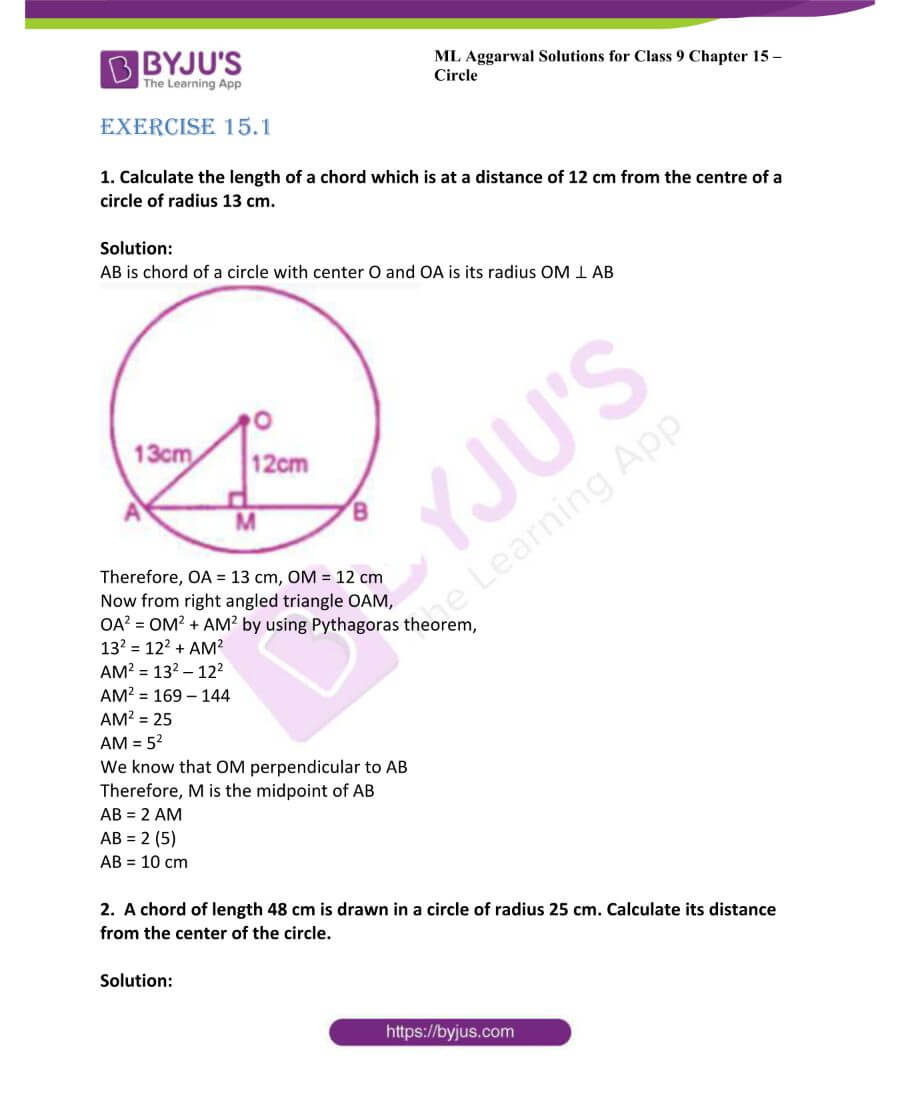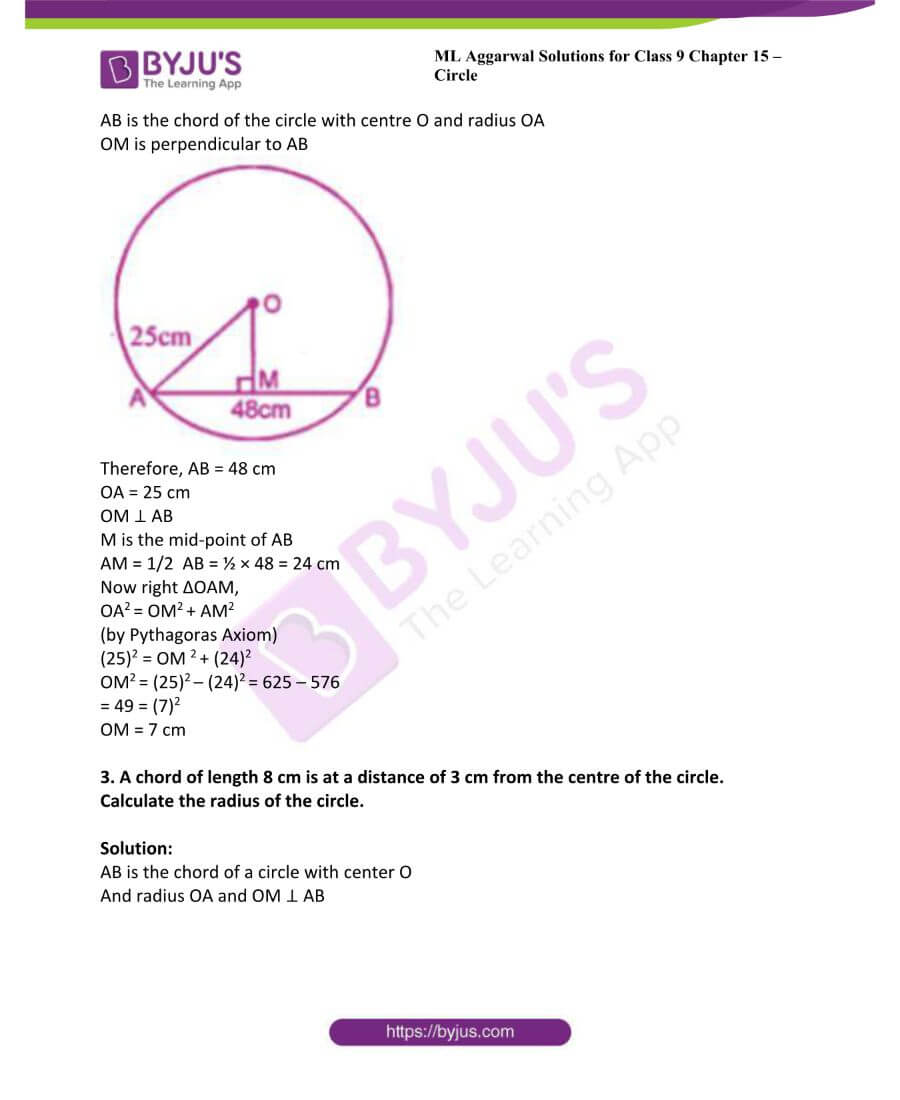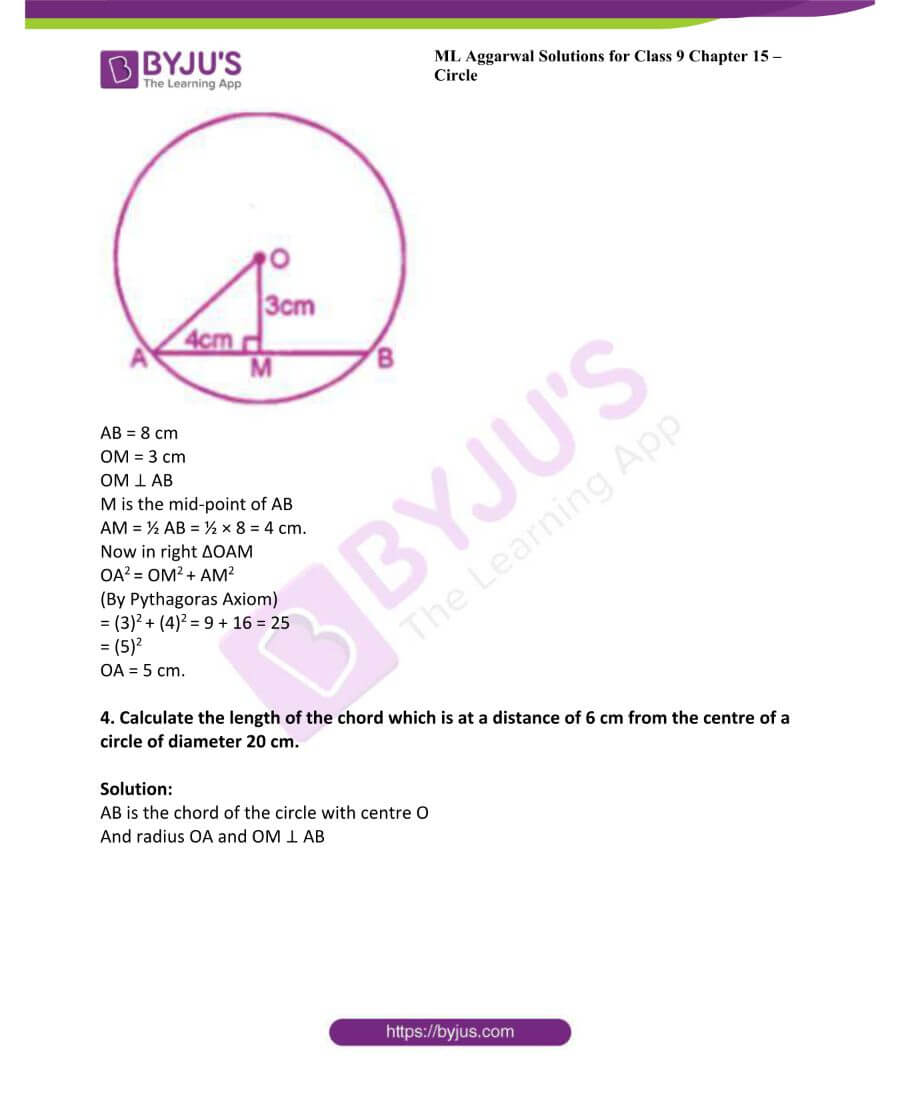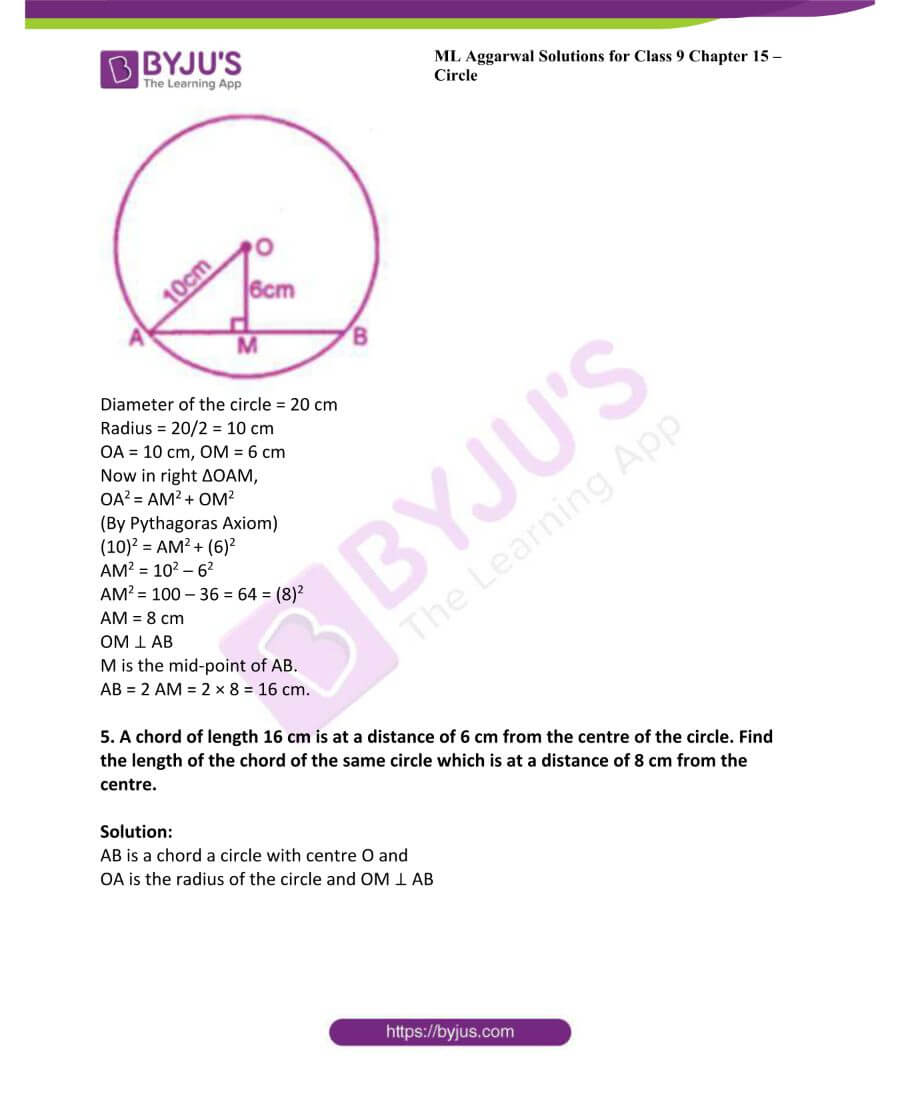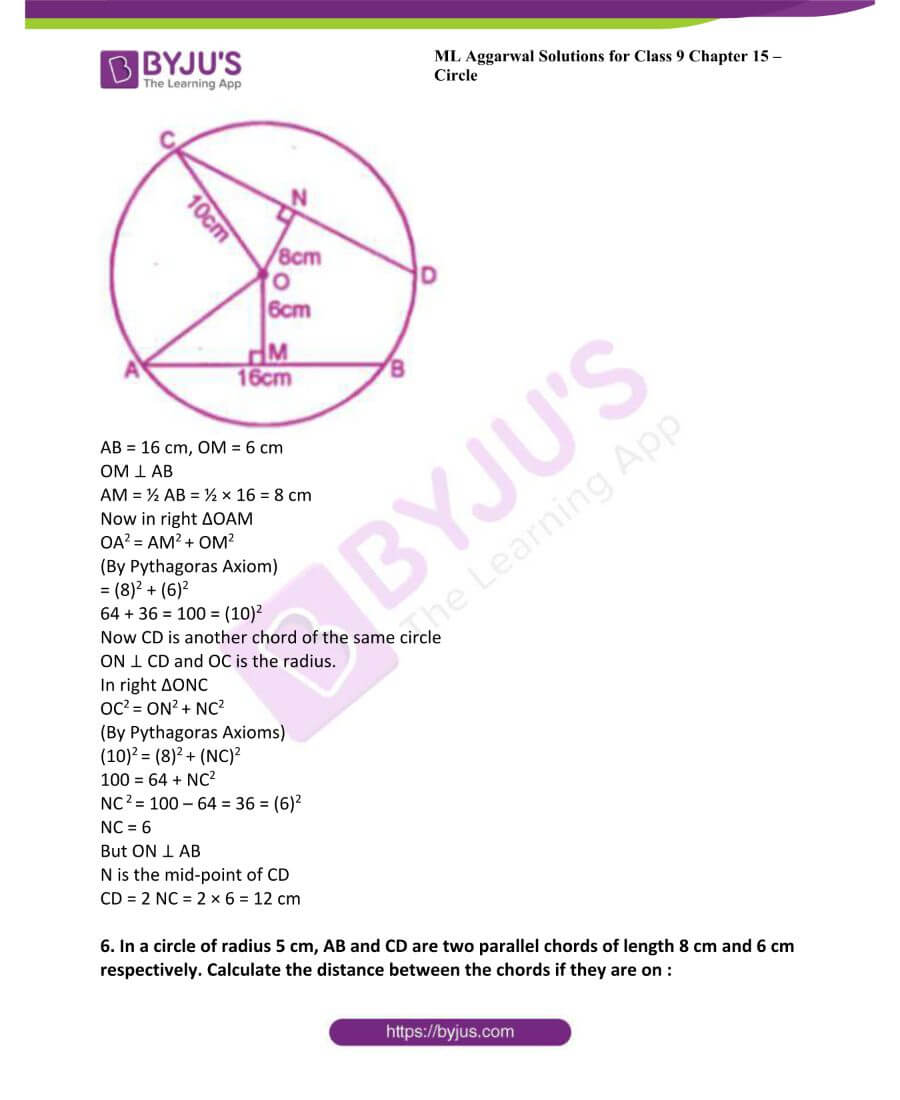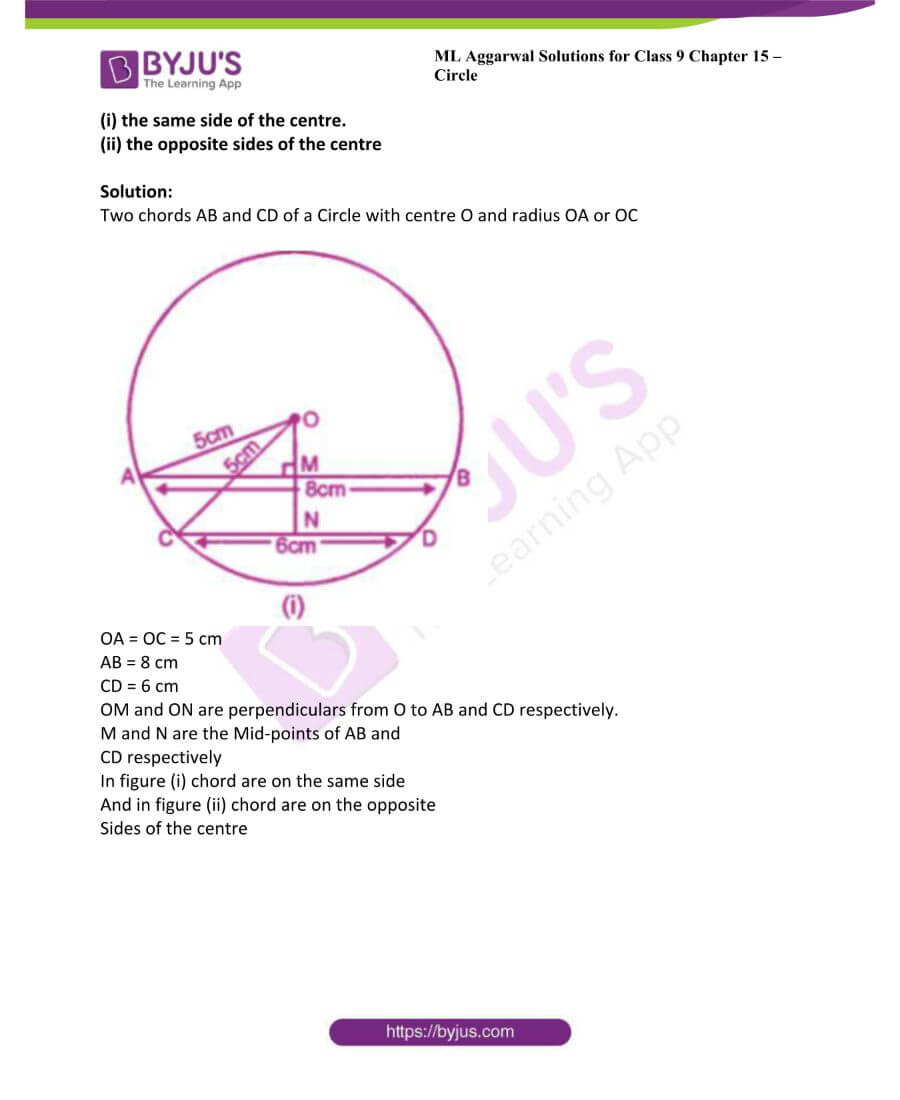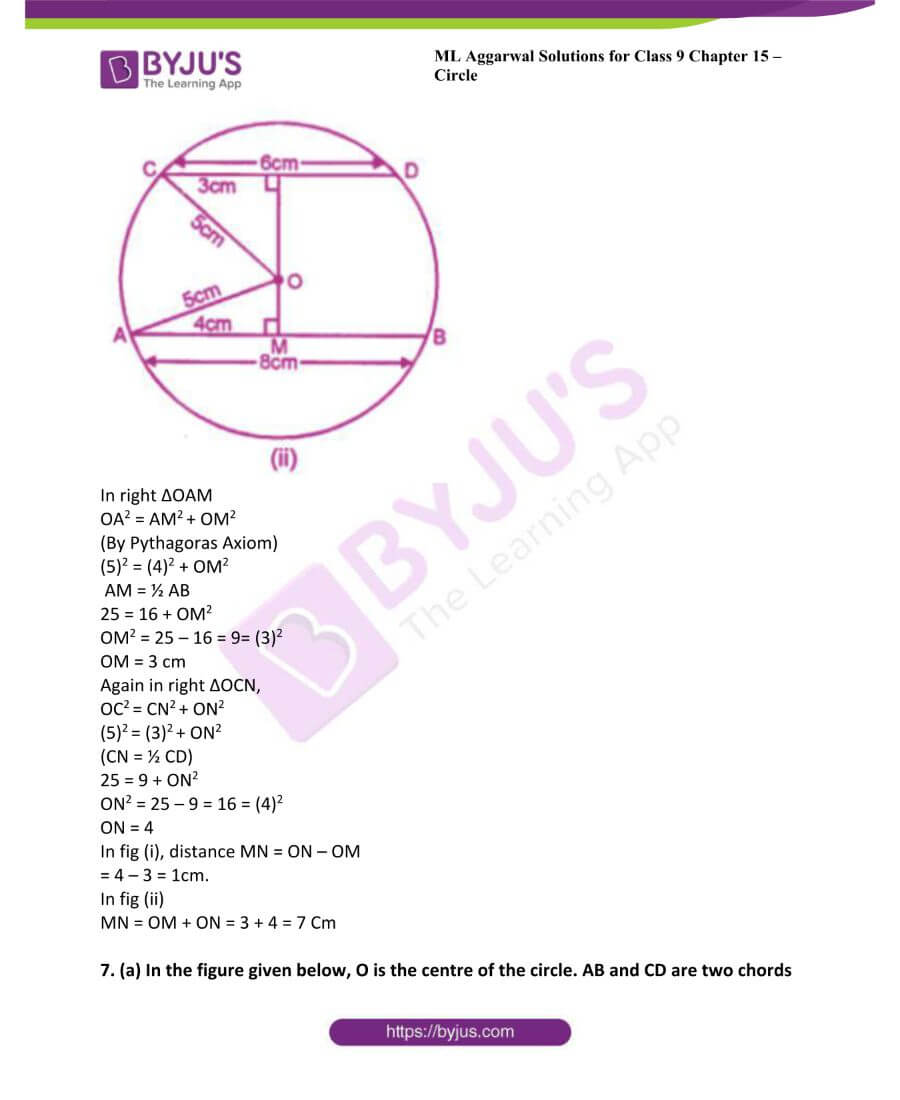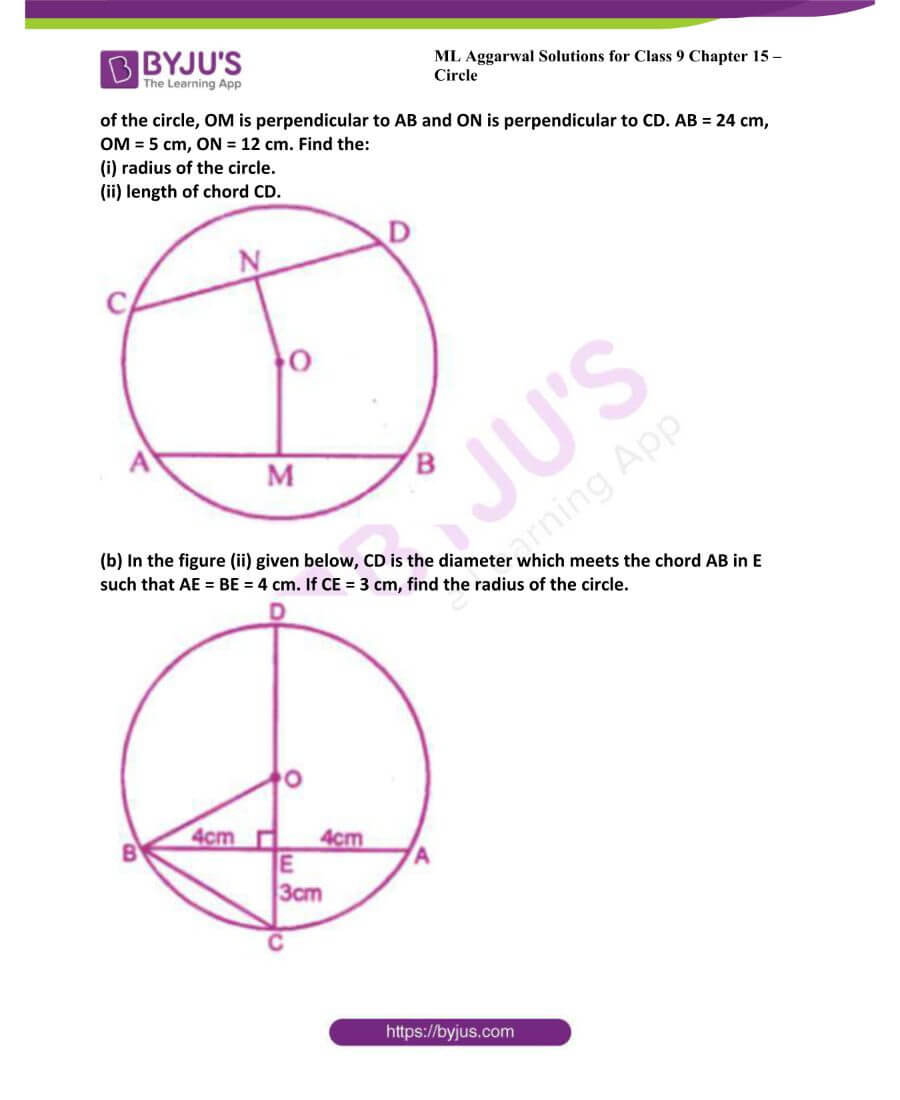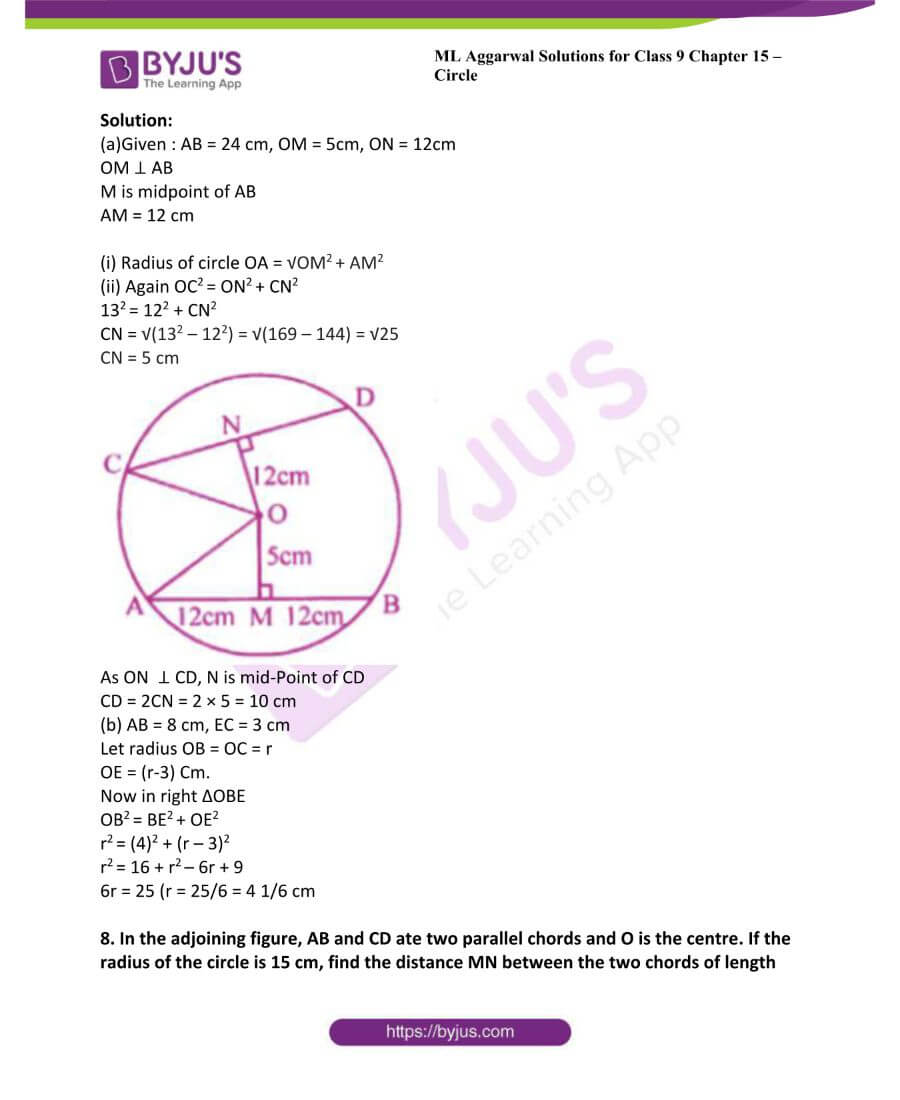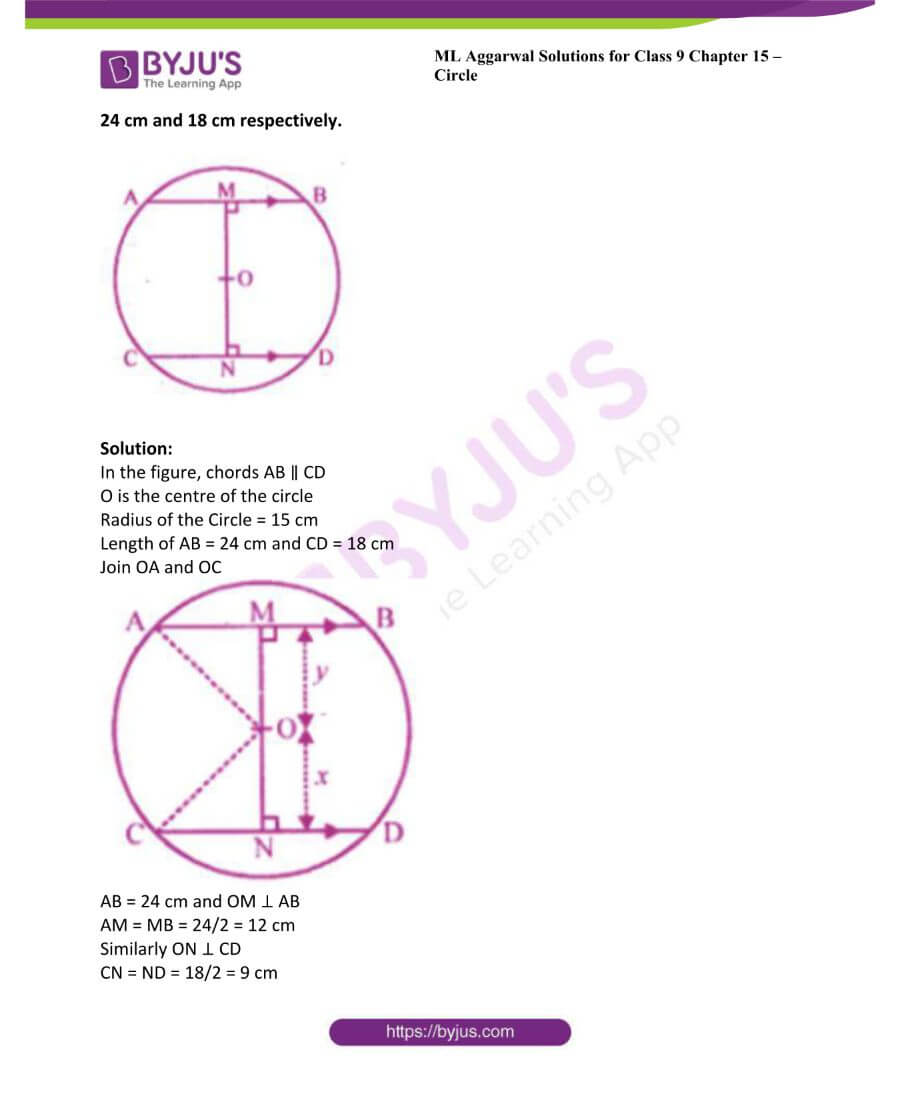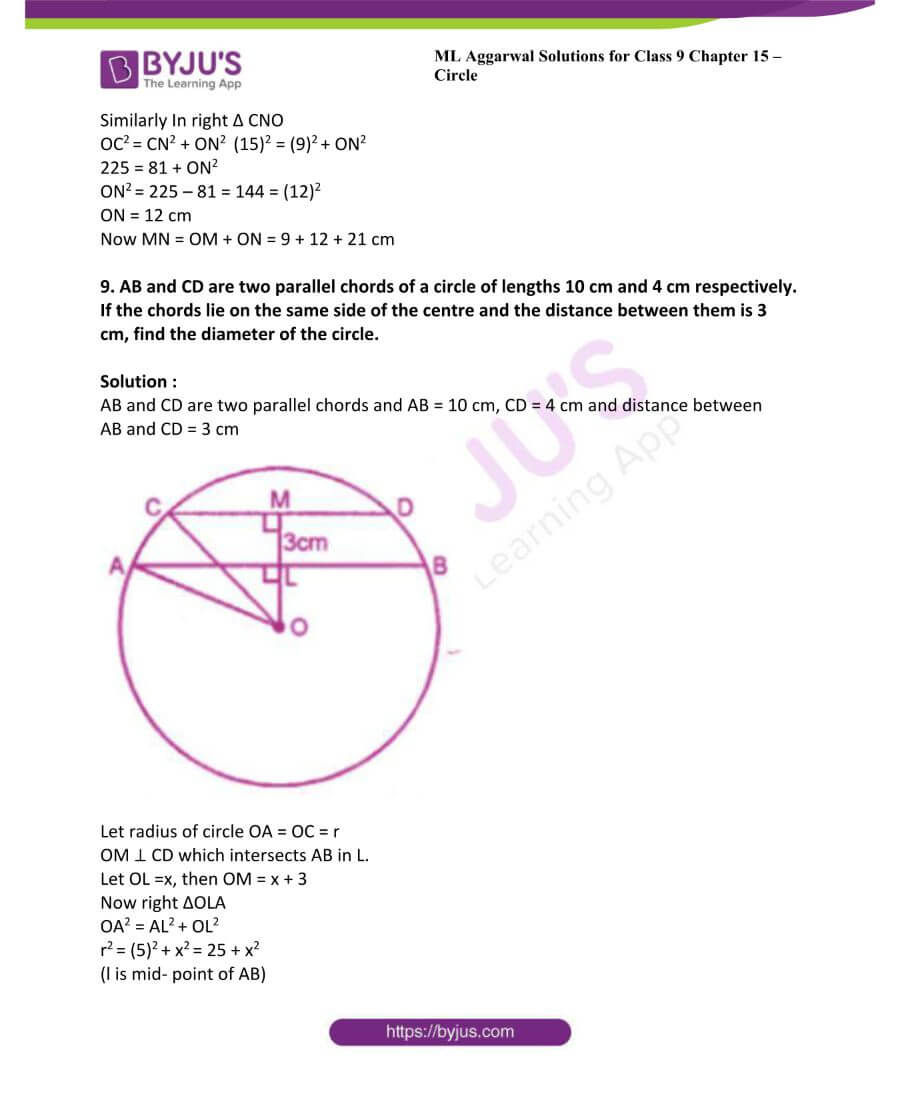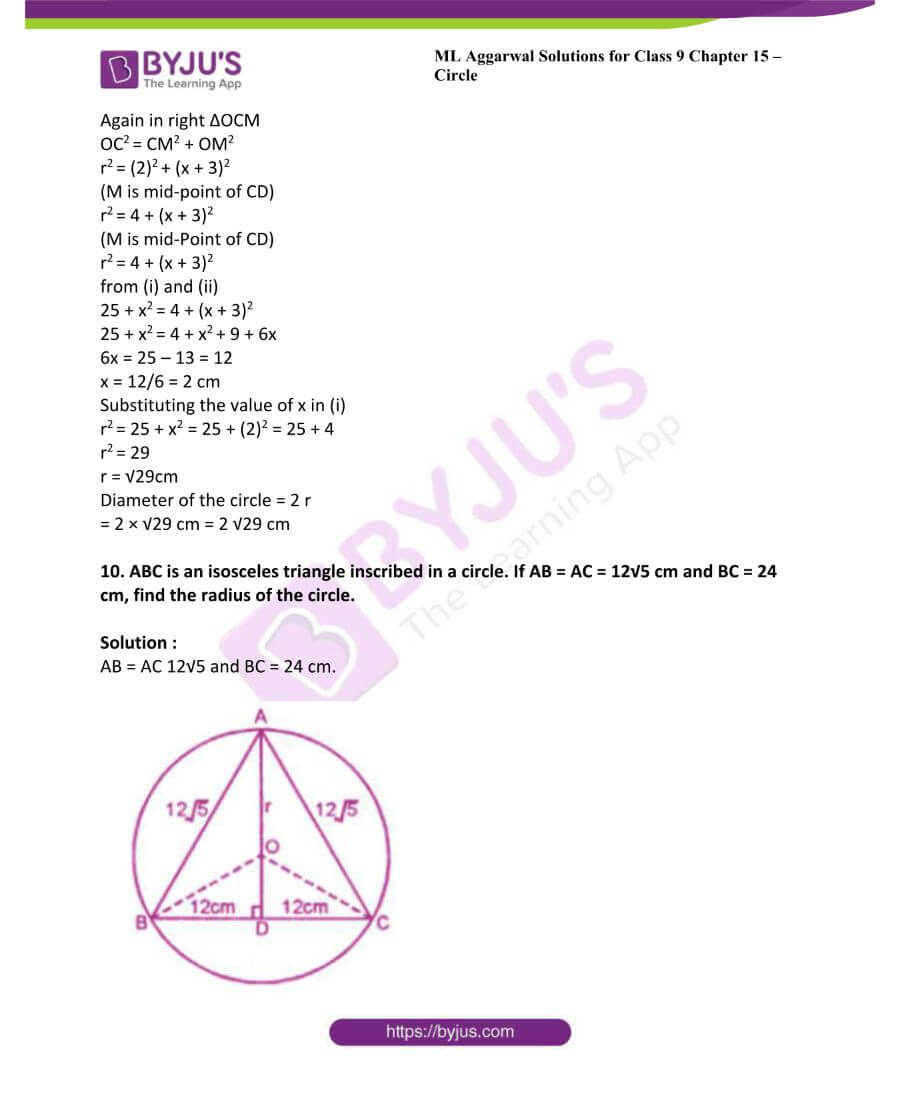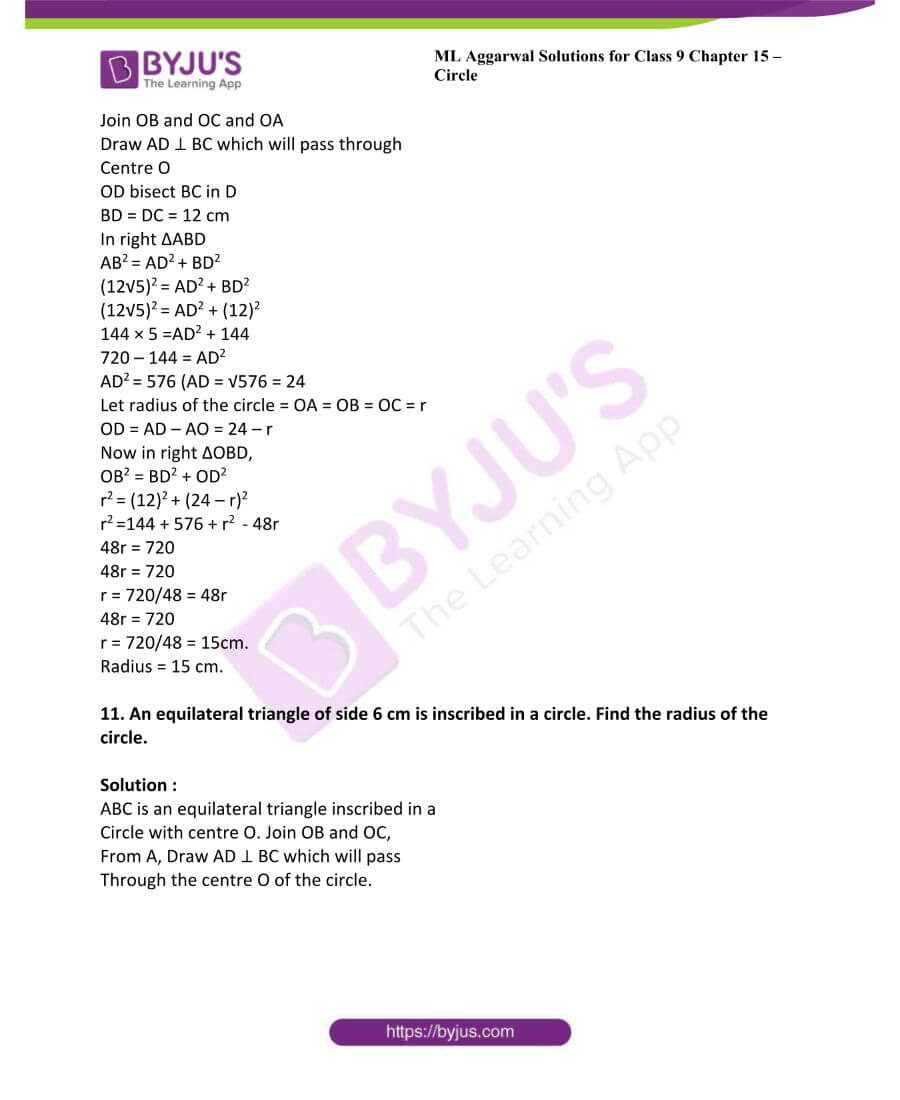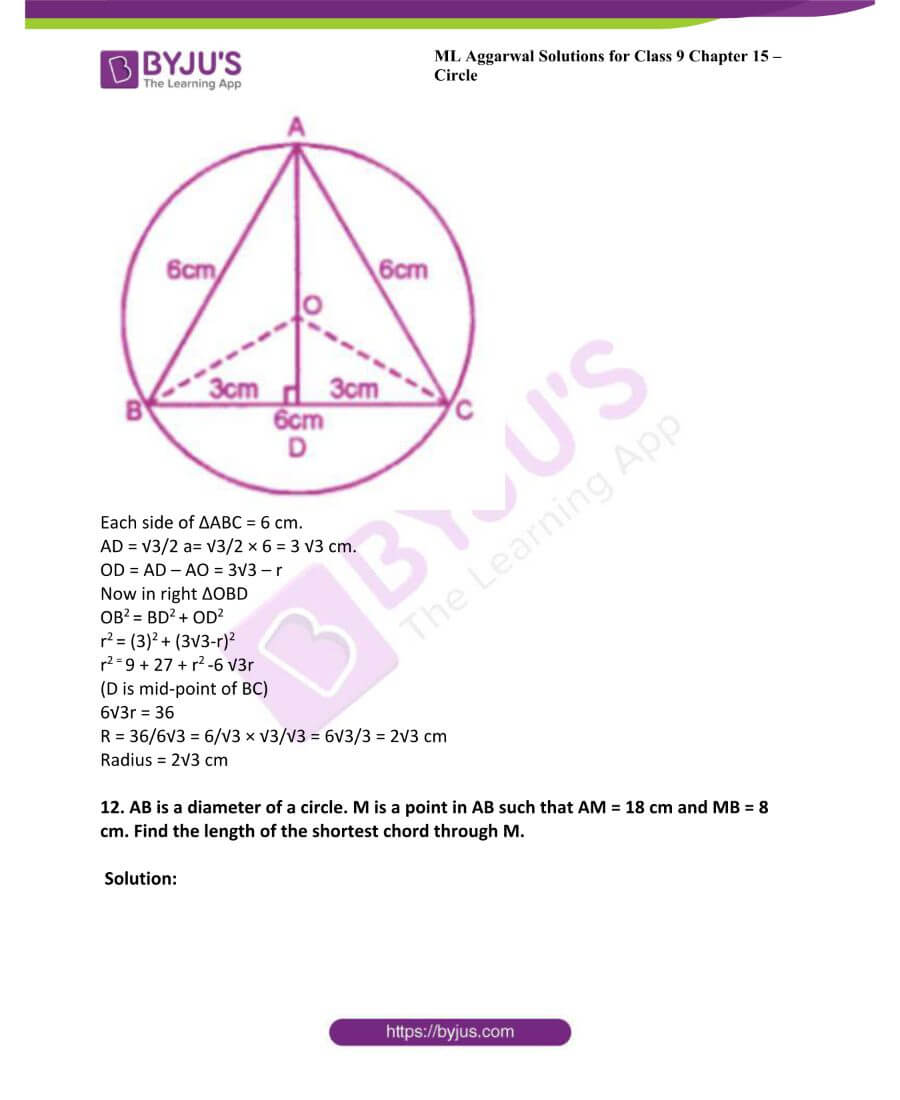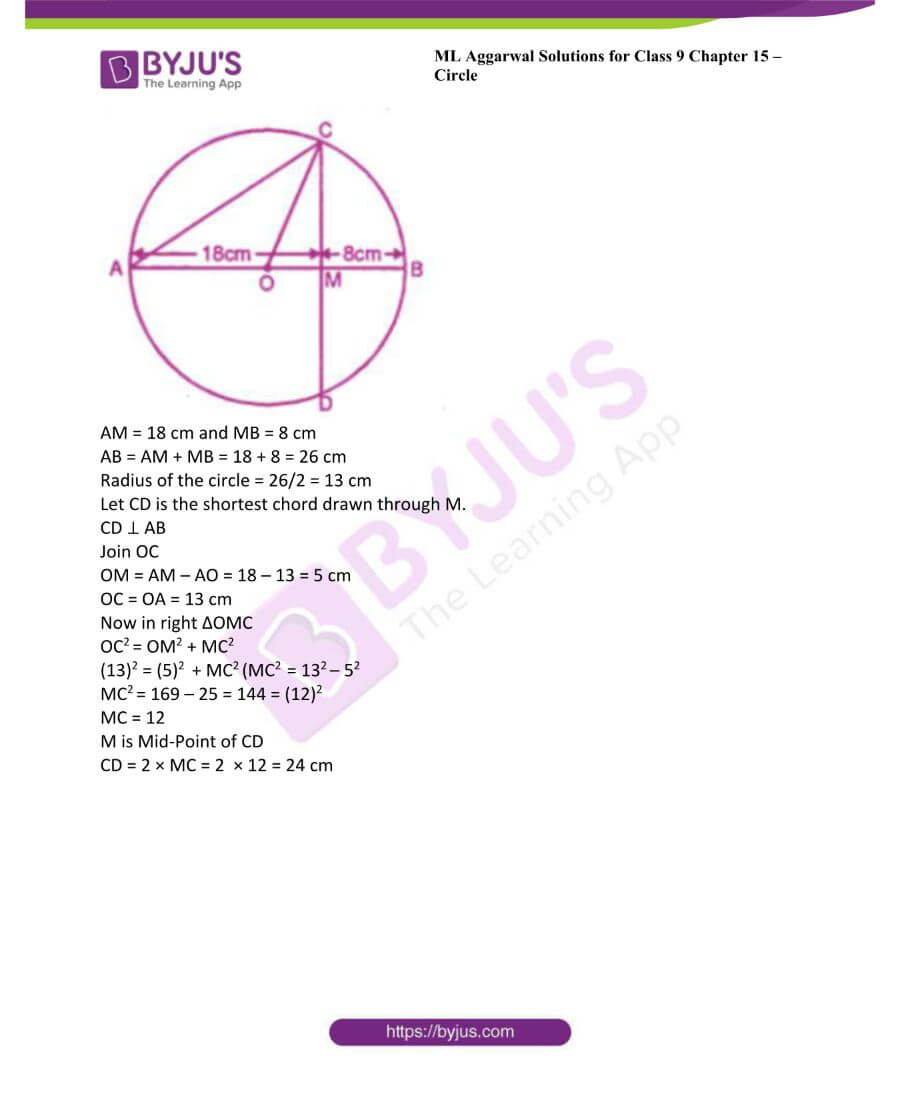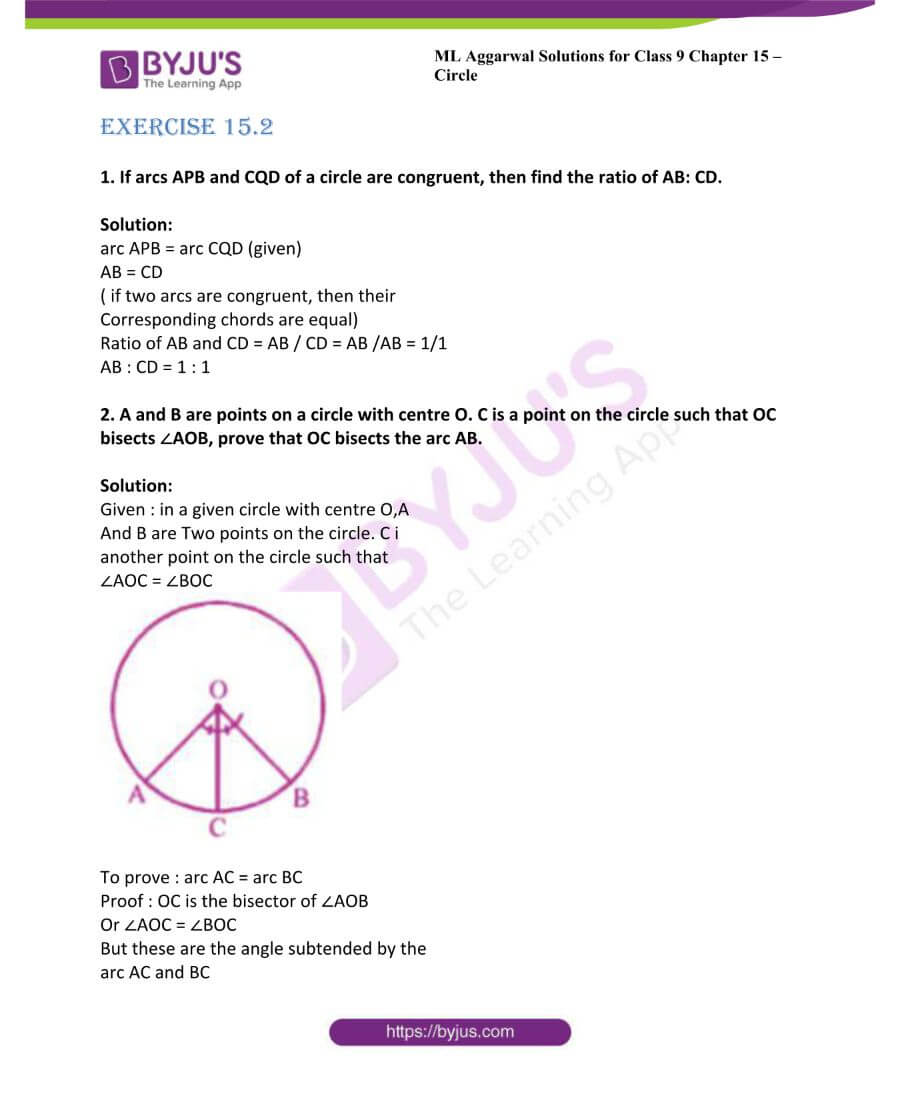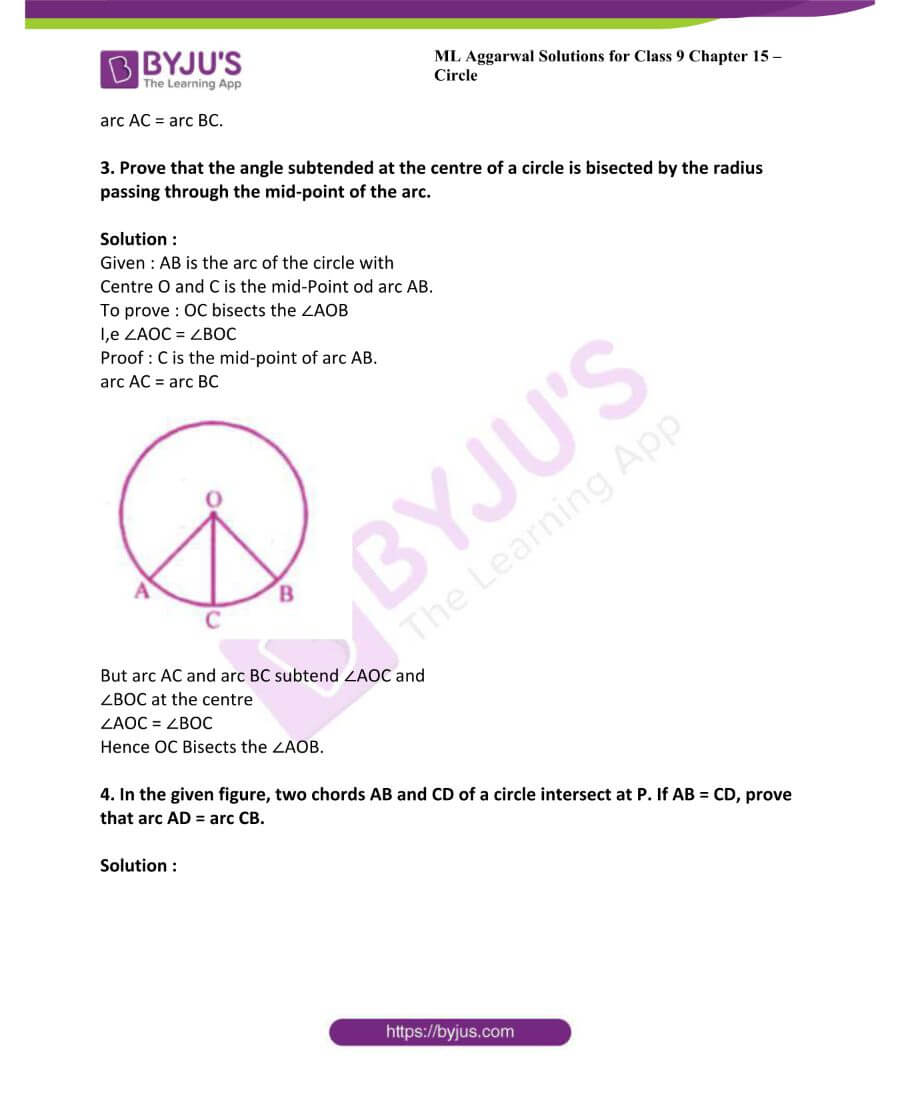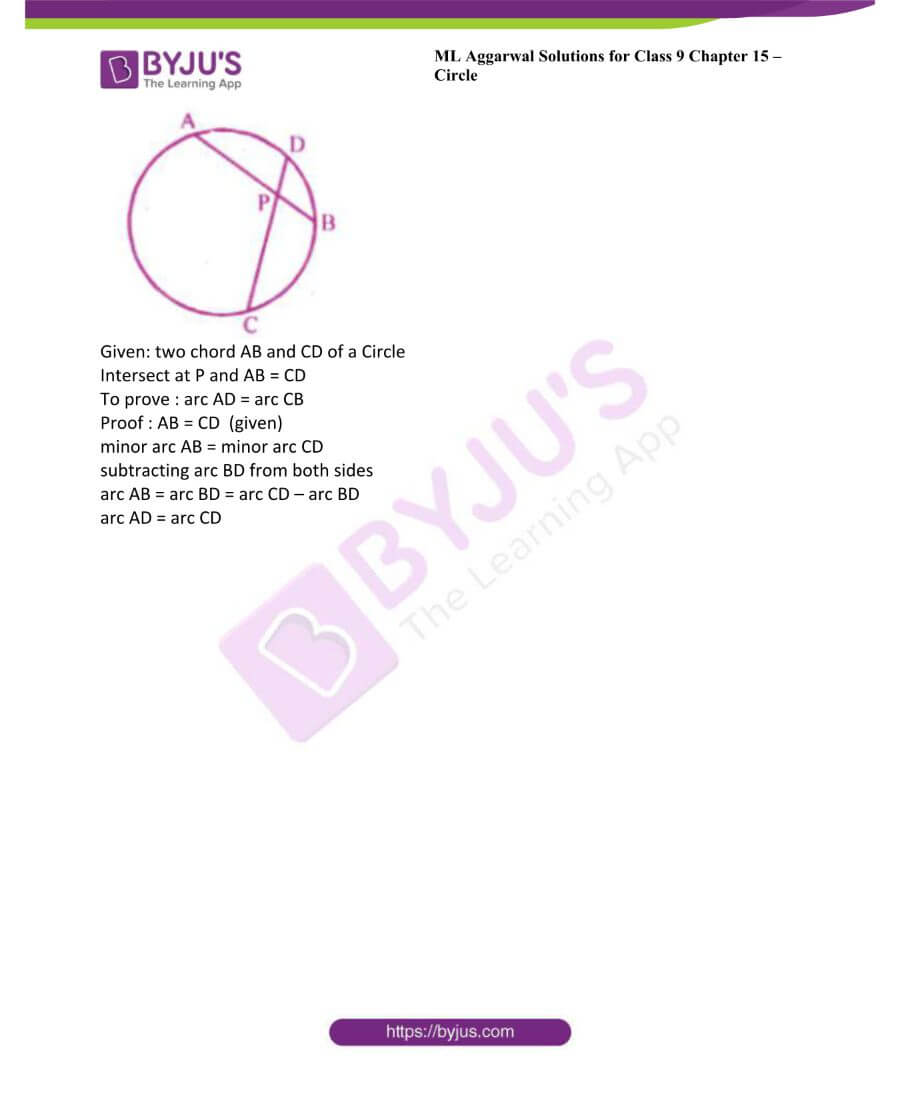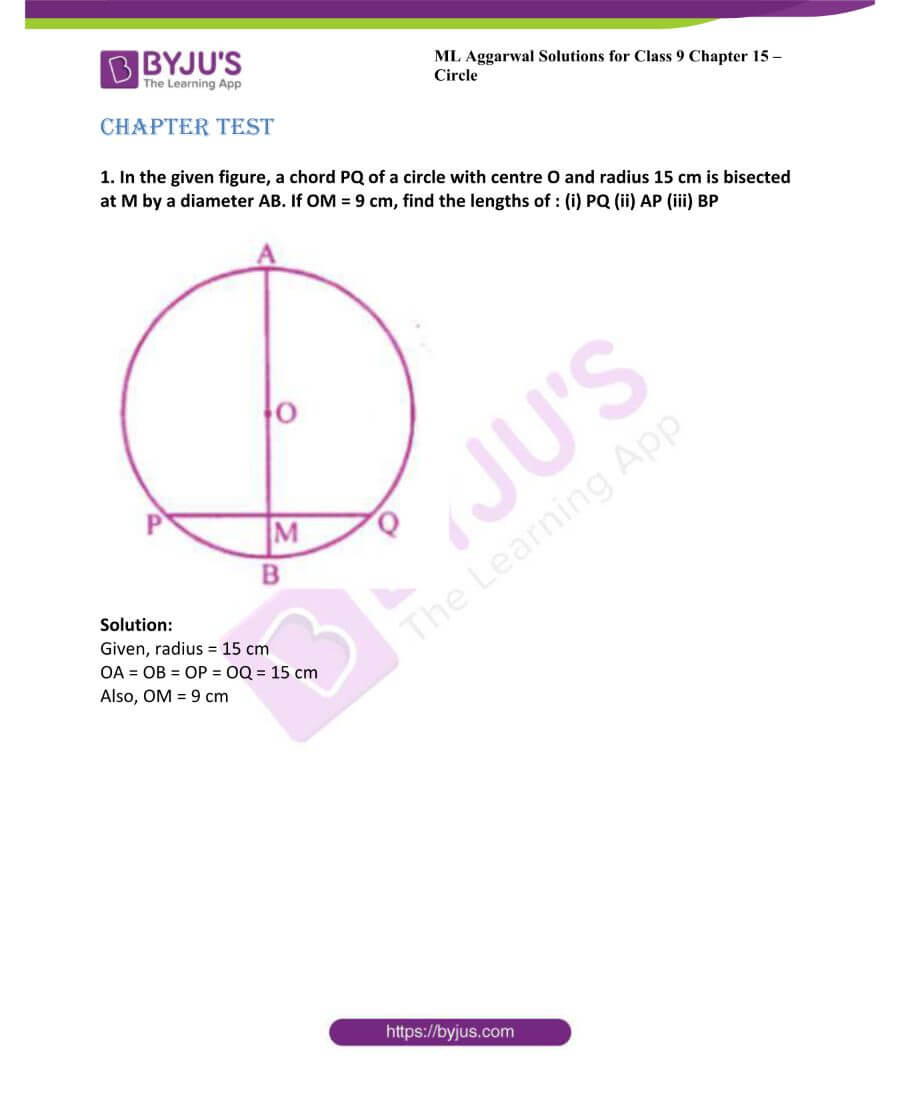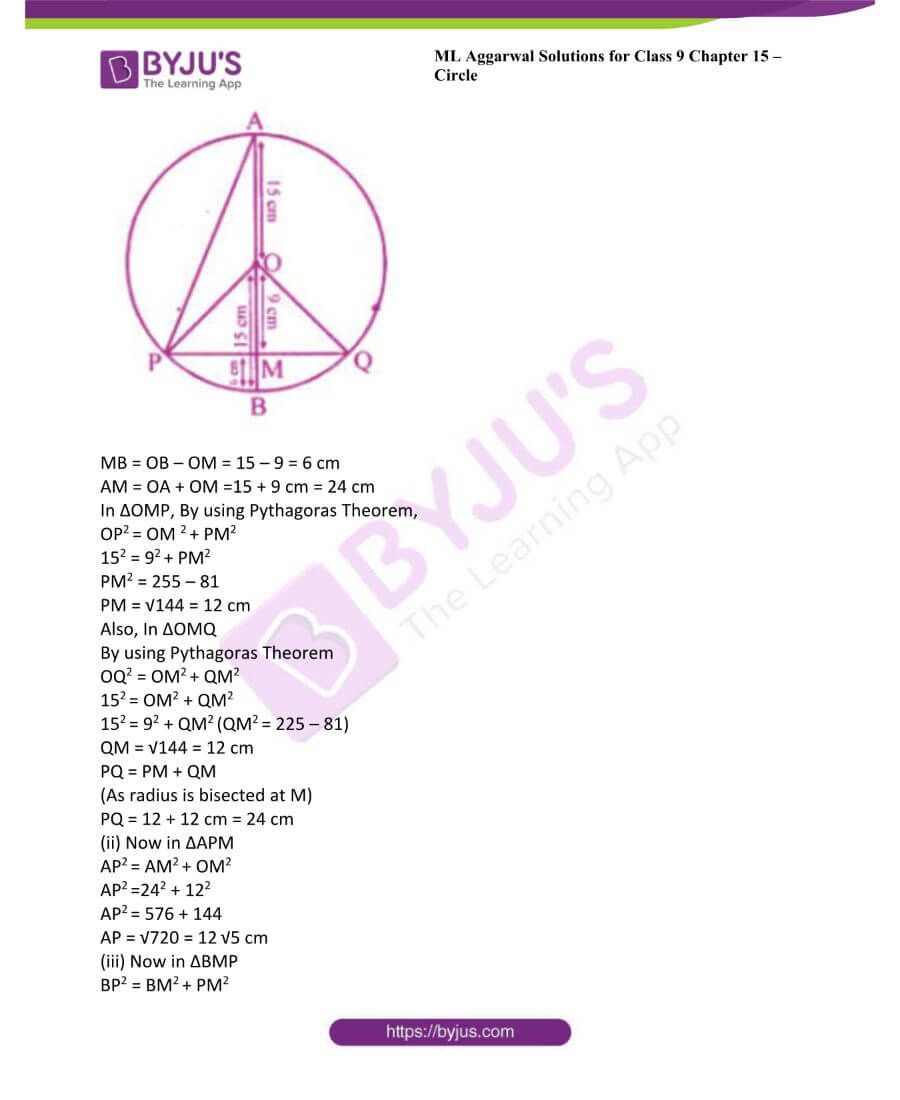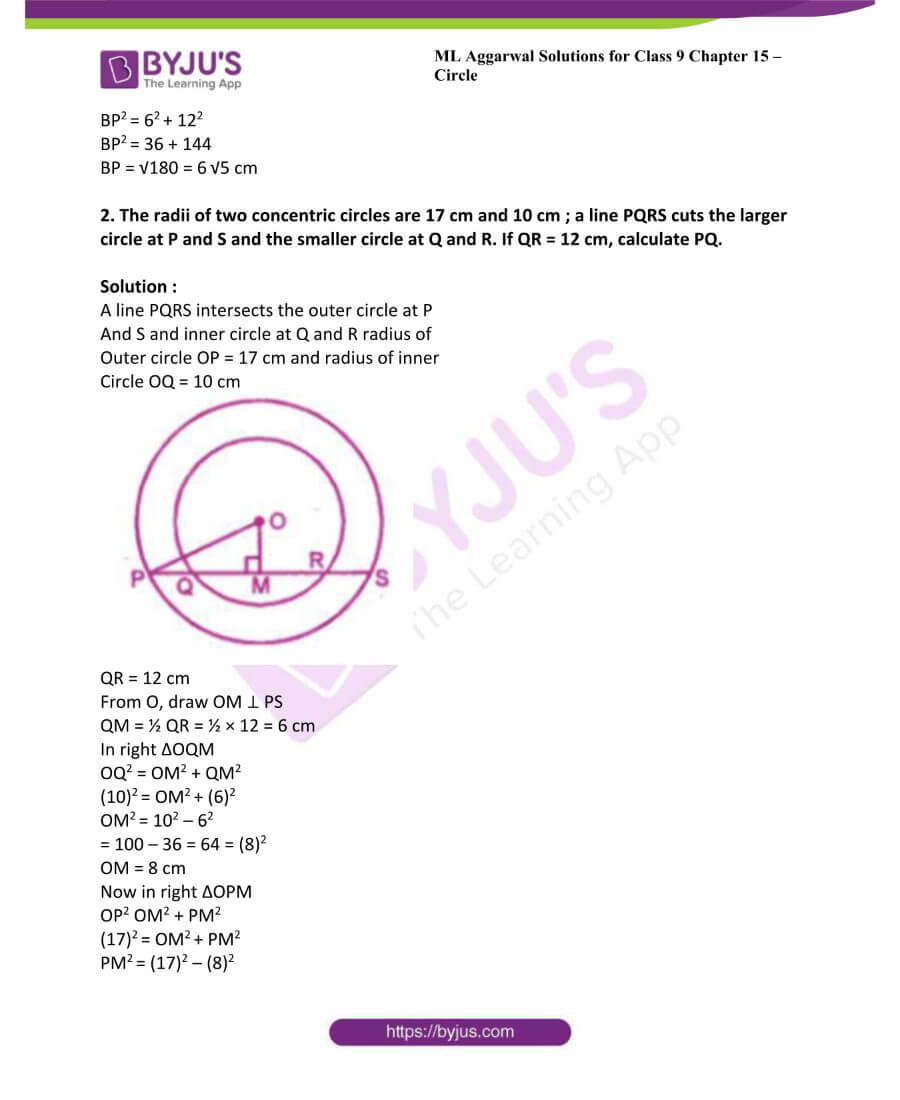### Access answers to ML Aggarwal Solutions for Class 9 Maths Chapter 15 – Circle

Exercise 15.1

1. Calculate the length of a chord which is at a distance of 12 cm from the centre of a circle of radius 13 cm.

Solution:

AB is chord of a circle with center O and OA is its radius OM ⊥ AB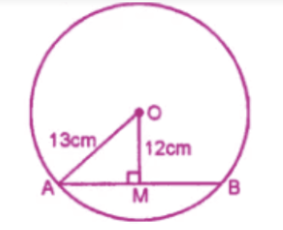Therefore, OA = 13 cm, OM = 12 cm

Now from right angled triangle OAM,

OA2 = OM2 + AM2 by using Pythagoras theorem,

132 = 122 + AM2

AM2 = 132 – 122

AM2 = 169 – 144

AM2 = 25

AM = 52

We know that OM perpendicular to AB

Therefore, M is the midpoint of AB

AB = 2 AM

AB = 2 (5)

AB = 10 cm

2. A chord of length 48 cm is drawn in a circle of radius 25 cm. Calculate its distance from the center of the circle.

Solution:

AB is the chord of the circle with centre O and radius OA

OM is perpendicular to AB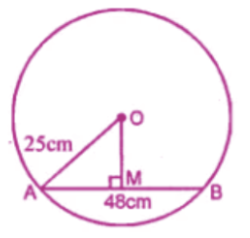Therefore, AB = 48 cm

OA = 25 cm

OM ⊥ AB

M is the mid-point of AB

AM = 1/2 AB = ½ × 48 = 24 cm

Now right ∆OAM,

OA2 = OM2 + AM2

(by Pythagoras Axiom)

(25)2 = OM 2 + (24)2

OM2 = (25)2 – (24)2 = 625 – 576

= 49 = (7)2

OM = 7 cm

3. A chord of length 8 cm is at a distance of 3 cm from the centre of the circle. Calculate the radius of the circle.

Solution:

AB is the chord of a circle with center O

And radius OA and OM ⊥ AB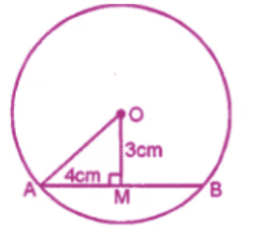AB = 8 cm

OM = 3 cm

OM ⊥ AB

M is the mid-point of AB

AM = ½ AB = ½ × 8 = 4 cm.

Now in right ∆OAM

OA2 = OM2 + AM2

(By Pythagoras Axiom)

= (3)2 + (4)2 = 9 + 16 = 25

= (5)2

OA = 5 cm.

4. Calculate the length of the chord which is at a distance of 6 cm from the centre of a circle of diameter 20 cm.

Solution:

AB is the chord of the circle with centre O

And radius OA and OM ⊥ AB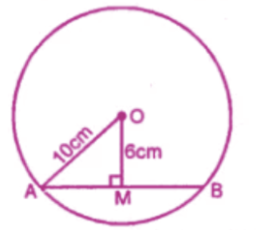Diameter of the circle = 20 cm

Radius = 20/2 = 10 cm

OA = 10 cm, OM = 6 cm

Now in right ∆OAM,

OA2 = AM2 + OM2

(By Pythagoras Axiom)

(10)2 = AM2 + (6)2

AM2 = 102 – 62

AM2 = 100 – 36 = 64 = (8)2

AM = 8 cm

OM ⊥ AB

M is the mid-point of AB.

AB = 2 AM = 2 × 8 = 16 cm.

5. A chord of length 16 cm is at a distance of 6 cm from the centre of the circle. Find the length of the chord of the same circle which is at a distance of 8 cm from the centre.

Solution:

AB is a chord a circle with centre O and

OA is the radius of the circle and OM ⊥ AB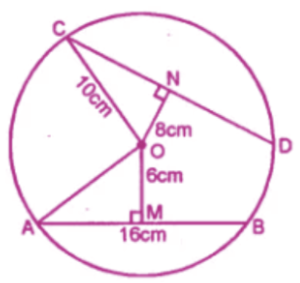AB = 16 cm, OM = 6 cm

OM ⊥ AB

AM = ½ AB = ½ × 16 = 8 cm

Now in right ∆OAM

OA2 = AM2 + OM2

(By Pythagoras Axiom)

= (8)2 + (6)2

64 + 36 = 100 = (10)2

Now CD is another chord of the same circle

ON ⊥ CD and OC is the radius.

In right ∆ONC

OC2 = ON2 + NC2

(By Pythagoras Axioms)

(10)2 = (8)2 + (NC)2

100 = 64 + NC2

NC 2 = 100 – 64 = 36 = (6)2

NC = 6

But ON ⊥ AB

N is the mid-point of CD

CD = 2 NC = 2 × 6 = 12 cm

6. In a circle of radius 5 cm, AB and CD are two parallel chords of length 8 cm and 6 cm respectively. Calculate the distance between the chords if they are on :

(i) the same side of the centre.

(ii) the opposite sides of the centre

Solution:

Two chords AB and CD of a Circle with centre O and radius OA or OC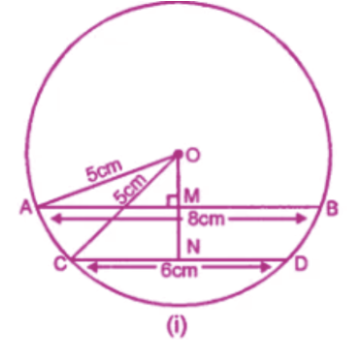OA = OC = 5 cm

AB = 8 cm

CD = 6 cm

OM and ON are perpendiculars from O to AB and CD respectively.

M and N are the Mid-points of AB and

CD respectively

In figure (i) chord are on the same side

And in figure (ii) chord are on the opposite

Sides of the centre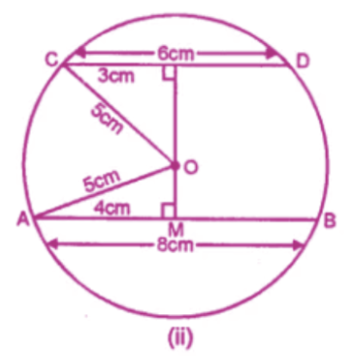In right ∆OAM

OA2 = AM2 + OM2

(By Pythagoras Axiom)

(5)2 = (4)2 + OM2

AM = ½ AB

25 = 16 + OM2

OM2 = 25 – 16 = 9= (3)2

OM = 3 cm

Again in right ∆OCN,

OC2 = CN2 + ON2

(5)2 = (3)2 + ON2

(CN = ½ CD)

25 = 9 + ON2

ON2 = 25 – 9 = 16 = (4)2

ON = 4

In fig (i), distance MN = ON – OM

= 4 – 3 = 1cm.

In fig (ii)

MN = OM + ON = 3 + 4 = 7 Cm

7. (a) In the figure given below, O is the centre of the circle. AB and CD are two chords of the circle, OM is perpendicular to AB and ON is perpendicular to CD. AB = 24 cm, OM = 5 cm, ON = 12 cm. Find the:

(ii) length of chord CD.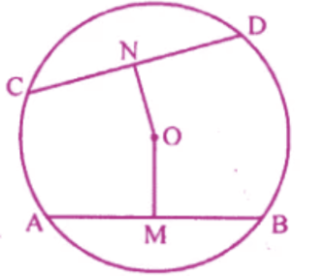(b) In the figure (ii) given below, CD is the diameter which meets the chord AB in E such that AE = BE = 4 cm. If CE = 3 cm, find the radius of the circle.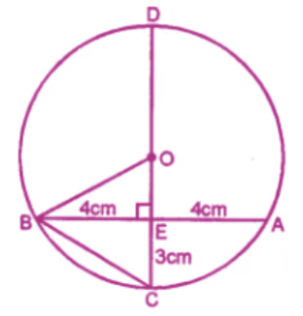Solution:

(a)Given : AB = 24 cm, OM = 5cm, ON = 12cm

OM ⊥ AB

M is midpoint of AB

AM = 12 cm

(i) Radius of circle OA = √OM2 + AM2

(ii) Again OC2 = ON2 + CN2

132 = 122 + CN2

CN = √(132 – 122) = √(169 – 144) = √25

CN = 5 cm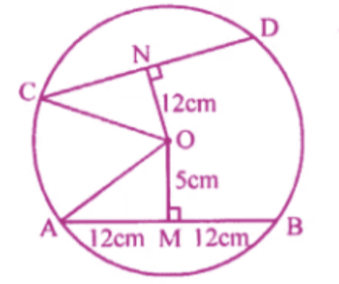As ON ⊥ CD, N is mid-Point of CD

CD = 2CN = 2 × 5 = 10 cm

(b) AB = 8 cm, EC = 3 cm

Let radius OB = OC = r

OE = (r-3) Cm.

Now in right ∆OBE

OB2 = BE2 + OE2

r2 = (4)2 + (r – 3)2

r2 = 16 + r2 – 6r + 9

6r = 25 (r = 25/6 = 4 1/6 cm

8. In the adjoining figure, AB and CD ate two parallel chords and O is the centre. If the radius of the circle is 15 cm, find the distance MN between the two chords of length 24 cm and 18 cm respectively.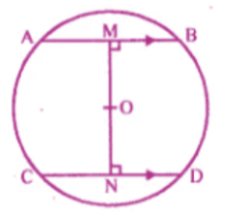Solution:

In the figure, chords AB ∥ CD

O is the centre of the circle

Radius of the Circle = 15 cm

Length of AB = 24 cm and CD = 18 cm

Join OA and OC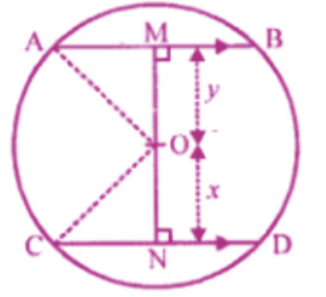AB = 24 cm and OM ⊥ AB

AM = MB = 24/2 = 12 cm

Similarly ON ⊥ CD

CN = ND = 18/2 = 9 cm

Similarly In right ∆ CNO

OC2 = CN2 + ON2 (15)2 = (9)2 + ON2

225 = 81 + ON2

ON2 = 225 – 81 = 144 = (12)2

ON = 12 cm

Now MN = OM + ON = 9 + 12 + 21 cm

9. AB and CD are two parallel chords of a circle of lengths 10 cm and 4 cm respectively. If the chords lie on the same side of the centre and the distance between them is 3 cm, find the diameter of the circle.

Solution :

AB and CD are two parallel chords and AB = 10 cm, CD = 4 cm and distance between

AB and CD = 3 cm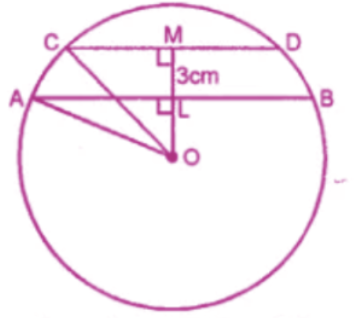Let radius of circle OA = OC = r

OM ⊥ CD which intersects AB in L.

Let OL =x, then OM = x + 3

Now right ∆OLA

OA2 = AL2 + OL2

r2 = (5)2 + x2 = 25 + x2

(l is mid- point of AB)

Again in right ∆OCM

OC2 = CM2 + OM2

r2 = (2)2 + (x + 3)2

(M is mid-point of CD)

r2 = 4 + (x + 3)2

(M is mid-Point of CD)

r2 = 4 + (x + 3)2

from (i) and (ii)

25 + x2 = 4 + (x + 3)2

25 + x2 = 4 + x2 + 9 + 6x

6x = 25 – 13 = 12

x = 12/6 = 2 cm

Substituting the value of x in (i)

r2 = 25 + x2 = 25 + (2)2 = 25 + 4

r2 = 29

r = √29cm

Diameter of the circle = 2 r

= 2 × √29 cm = 2 √29 cm

10. ABC is an isosceles triangle inscribed in a circle. If AB = AC = 12√5 cm and BC = 24 cm, find the radius of the circle.

Solution :

AB = AC 12√5 and BC = 24 cm.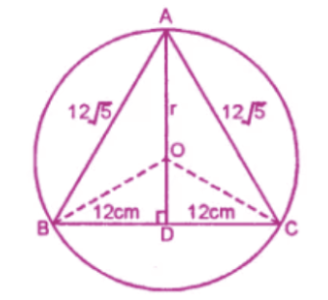Join OB and OC and OA

Draw AD ⊥ BC which will pass through

Centre O

OD bisect BC in D

BD = DC = 12 cm

In right ∆ABD

144 × 5 =AD2 + 144

Let radius of the circle = OA = OB = OC = r

OD = AD – AO = 24 – r

Now in right ∆OBD,

OB2 = BD2 + OD2

r2 = (12)2 + (24 – r)2

r2 =144 + 576 + r2 – 48r

48r = 720

48r = 720

r = 720/48 = 48r

48r = 720

r = 720/48 = 15cm.

11. An equilateral triangle of side 6 cm is inscribed in a circle. Find the radius of the circle.

Solution :

ABC is an equilateral triangle inscribed in a

Circle with centre O. Join OB and OC,

From A, Draw AD ⊥ BC which will pass

Through the centre O of the circle.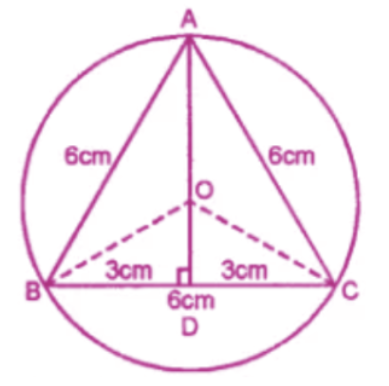Each side of ∆ABC = 6 cm.

AD = √3/2 a= √3/2 × 6 = 3 √3 cm.

OD = AD – AO = 3√3 – r

Now in right ∆OBD

OB2 = BD2 + OD2

r2 = (3)2 + (3√3-r)2

r2 = 9 + 27 + r2 -6 √3r

(D is mid-point of BC)

6√3r = 36

R = 36/6√3 = 6/√3 × √3/√3 = 6√3/3 = 2√3 cm

12. AB is a diameter of a circle. M is a point in AB such that AM = 18 cm and MB = 8 cm. Find the length of the shortest chord through M.

Solution: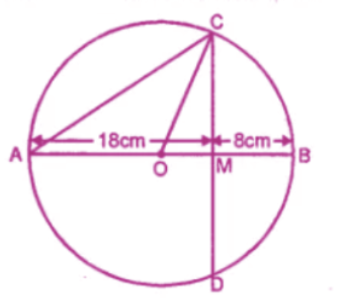AM = 18 cm and MB = 8 cm

AB = AM + MB = 18 + 8 = 26 cm

Radius of the circle = 26/2 = 13 cm

Let CD is the shortest chord drawn through M.

CD ⊥ AB

Join OC

OM = AM – AO = 18 – 13 = 5 cm

OC = OA = 13 cm

Now in right ∆OMC

OC2 = OM2 + MC2

(13)2 = (5)2 + MC2 (MC2 = 132 – 52

MC2 = 169 – 25 = 144 = (12)2

MC = 12

M is Mid-Point of CD

CD = 2 × MC = 2 × 12 = 24 cm

Exercise 15.2

1. If arcs APB and CQD of a circle are congruent, then find the ratio of AB: CD.

Solution:

arc APB = arc CQD (given)

AB = CD

( if two arcs are congruent, then their

Corresponding chords are equal)

Ratio of AB and CD = AB / CD = AB /AB = 1/1

AB : CD = 1 : 1

2. A and B are points on a circle with centre O. C is a point on the circle such that OC bisects ∠AOB, prove that OC bisects the arc AB.

Solution:

Given : in a given circle with centre O,A

And B are Two points on the circle. C i

another point on the circle such that

∠AOC = ∠BOC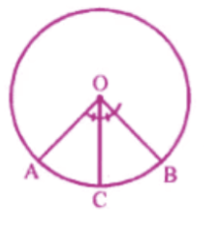To prove : arc AC = arc BC

Proof : OC is the bisector of ∠AOB

Or ∠AOC = ∠BOC

But these are the angle subtended by the

arc AC and BC

arc AC = arc BC.

3. Prove that the angle subtended at the centre of a circle is bisected by the radius passing through the mid-point of the arc.

Solution :

Given : AB is the arc of the circle with

Centre O and C is the mid-Point od arc AB.

To prove : OC bisects the ∠AOB

I,e ∠AOC = ∠BOC

Proof : C is the mid-point of arc AB.

arc AC = arc BC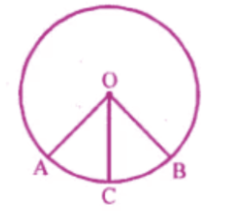But arc AC and arc BC subtend ∠AOC and

∠BOC at the centre

∠AOC = ∠BOC

Hence OC Bisects the ∠AOB.

4. In the given figure, two chords AB and CD of a circle intersect at P. If AB = CD, prove that arc AD = arc CB.

Solution :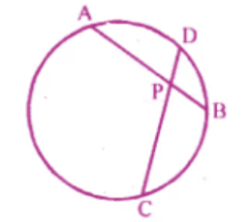Given: two chord AB and CD of a Circle

Intersect at P and AB = CD

To prove : arc AD = arc CB

Proof : AB = CD (given)

minor arc AB = minor arc CD

subtracting arc BD from both sides

arc AB = arc BD = arc CD – arc BD

Chapter test

1. In the given figure, a chord PQ of a circle with centre O and radius 15 cm is bisected at M by a diameter AB. If OM = 9 cm, find the lengths of : (i) PQ (ii) AP (iii) BP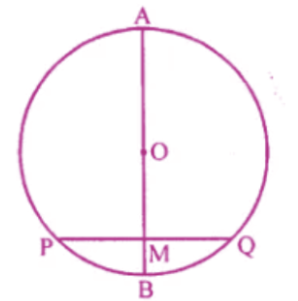Solution:

OA = OB = OP = OQ = 15 cm

Also, OM = 9 cm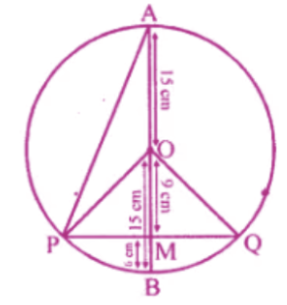MB = OB – OM = 15 – 9 = 6 cm

AM = OA + OM =15 + 9 cm = 24 cm

In ∆OMP, By using Pythagoras Theorem,

OP2 = OM 2 + PM2

152 = 92 + PM2

PM2 = 255 – 81

PM = √144 = 12 cm

Also, In ∆OMQ

By using Pythagoras Theorem

OQ2 = OM2 + QM2

152 = OM2 + QM2

152 = 92 + QM2 (QM2 = 225 – 81)

QM = √144 = 12 cm

PQ = PM + QM

(As radius is bisected at M)

PQ = 12 + 12 cm = 24 cm

(ii) Now in ∆APM

AP2 = AM2 + OM2

AP2 =242 + 122

AP2 = 576 + 144

AP = √720 = 12 √5 cm

(iii) Now in ∆BMP

BP2 = BM2 + PM2

BP2 = 62 + 122

BP2 = 36 + 144

BP = √180 = 6 √5 cm

2. The radii of two concentric circles are 17 cm and 10 cm ; a line PQRS cuts the larger circle at P and S and the smaller circle at Q and R. If QR = 12 cm, calculate PQ.

Solution :

A line PQRS intersects the outer circle at P

And S and inner circle at Q and R radius of

Outer circle OP = 17 cm and radius of inner

Circle OQ = 10 cm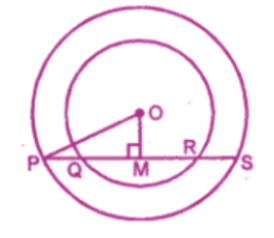QR = 12 cm

From O, draw OM ⊥ PS

QM = ½ QR = ½ × 12 = 6 cm

In right ∆OQM

OQ2 = OM2 + QM2

(10)2 = OM2 + (6)2

OM2 = 102 – 62

= 100 – 36 = 64 = (8)2

OM = 8 cm

Now in right ∆OPM

OP2 OM2 + PM2

(17)2 = OM2 + PM2

PM2 = (17)2 – (8)2

= 289 – 64 = 225 = (15)2

PM = 15 cm

PQ = PM – QM = 15 – 6 = 9 cm